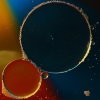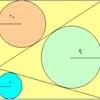Search by Topic

Resources tagged with Regular polygons and circles similar to Chord:

Filter by: Content type:
Age range:
Challenge level:

There are 67 results

Broad Topics > Angles, Polygons, and Geometrical Proof > Regular polygons and circlesThe Pillar of Chios

Age 14 to 16 Challenge Level:

Semicircles are drawn on the sides of a rectangle. Prove that the sum of the areas of the four crescents is equal to the area of the rectangle.Fitting In

Age 14 to 16 Challenge Level:

The largest square which fits into a circle is ABCD and EFGH is a square with G and H on the line CD and E and F on the circumference of the circle. Show that AB = 5EF. Similarly the largest. . . .Just Touching

Age 16 to 18 Challenge Level:

Three semi-circles have a common diameter, each touches the other two and two lie inside the biggest one. What is the radius of the circle that touches all three semi-circles?Circles in Circles

Age 16 to 18 Challenge Level:

This pattern of six circles contains three unit circles. Work out the radii of the other three circles and the relationship between them.Semi-detached

Age 14 to 16 Challenge Level:

A square of area 40 square cms is inscribed in a semicircle. Find the area of the square that could be inscribed in a circle of the same radius.Some(?) of the Parts

Age 14 to 16 Challenge Level:

A circle touches the lines OA, OB and AB where OA and OB are perpendicular. Show that the diameter of the circle is equal to the perimeter of the trianglePolycircles

Age 14 to 16 Challenge Level:

Show that for any triangle it is always possible to construct 3 touching circles with centres at the vertices. Is it possible to construct touching circles centred at the vertices of any polygon?Orthogonal Circle

Age 16 to 18 Challenge Level:

Given any three non intersecting circles in the plane find another circle or straight line which cuts all three circles orthogonally.Semi-square

Age 14 to 16 Challenge Level:

What is the ratio of the area of a square inscribed in a semicircle to the area of the square inscribed in the entire circle?Three Four Five

Age 14 to 16 Challenge Level:

Two semi-circles (each of radius 1/2) touch each other, and a semi-circle of radius 1 touches both of them. Find the radius of the circle which touches all three semi-circles.Logosquares

Age 16 to 18 Challenge Level:

Ten squares form regular rings either with adjacent or opposite vertices touching. Calculate the inner and outer radii of the rings that surround the squares.Pent

Age 14 to 18 Challenge Level:

The diagram shows a regular pentagon with sides of unit length. Find all the angles in the diagram. Prove that the quadrilateral shown in red is a rhombus.Incircles Explained

Age 16 to 18Age 14 to 16 Challenge Level:

The sides of a triangle are 25, 39 and 40 units of length. Find the diameter of the circumscribed circle.Retracircles

Age 16 to 18 Challenge Level:

Four circles all touch each other and a circumscribing circle. Find the ratios of the radii and prove that joining 3 centres gives a 3-4-5 triangle.Age 14 to 16 Challenge Level:

Given a square ABCD of sides 10 cm, and using the corners as centres, construct four quadrants with radius 10 cm each inside the square. The four arcs intersect at P, Q, R and S. Find the. . . .Encircling

Age 14 to 16 Challenge Level:

An equilateral triangle is sitting on top of a square. What is the radius of the circle that circumscribes this shape?Curvy Areas

Age 14 to 16 Challenge Level:

Have a go at creating these images based on circles. What do you notice about the areas of the different sections?Ball Bearings

Age 16 to 18 Challenge Level:

If a is the radius of the axle, b the radius of each ball-bearing, and c the radius of the hub, why does the number of ball bearings n determine the ratio c/a? Find a formula for c/a in terms of n.Circle Packing

Age 14 to 16 Challenge Level:

Equal circles can be arranged so that each circle touches four or six others. What percentage of the plane is covered by circles in each packing pattern? ...Partly Circles

Age 14 to 16 Challenge Level:

What is the same and what is different about these circle questions? What connections can you make?Salinon

Age 14 to 16 Challenge Level:

This shape comprises four semi-circles. What is the relationship between the area of the shaded region and the area of the circle on AB as diameter?Escriptions

Age 16 to 18 Challenge Level:

For any right-angled triangle find the radii of the three escribed circles touching the sides of the triangle externally.Rolling Coins

Age 14 to 16 Challenge Level:

A blue coin rolls round two yellow coins which touch. The coins are the same size. How many revolutions does the blue coin make when it rolls all the way round the yellow coins? Investigate for a. . . .The Medieval Octagon

Age 14 to 16 Challenge Level:

Medieval stonemasons used a method to construct octagons using ruler and compasses... Is the octagon regular? Proof please.Squaring the Circle and Circling the Square

Age 14 to 16 Challenge Level:

If you continue the pattern, can you predict what each of the following areas will be? Try to explain your prediction.LOGO Challenge - Circles as Animals

Age 11 to 16 Challenge Level:

See if you can anticipate successive 'generations' of the two animals shown here.Age 14 to 16 Challenge Level:

Investigate the properties of quadrilaterals which can be drawn with a circle just touching each side and another circle just touching each vertex.Area I'n It

Age 16 to 18 Challenge Level:

Triangle ABC has altitudes h1, h2 and h3. The radius of the inscribed circle is r, while the radii of the escribed circles are r1, r2 and r3 respectively. Prove: 1/r = 1/h1 + 1/h2 + 1/h3 = 1/r1 +. . . .Ford Circles

Age 16 to 18 Challenge Level:

Can you find the link between these beautiful circle patterns and Farey Sequences?A Rational Search

Age 14 to 18 Challenge Level:

Investigate constructible images which contain rational areas.Lunar Angles

Age 16 to 18 Challenge Level:

What is the sum of the angles of a triangle whose sides are circular arcs on a flat surface? What if the triangle is on the surface of a sphere?Kissing

Age 16 to 18 Challenge Level:

Two perpendicular lines are tangential to two identical circles that touch. What is the largest circle that can be placed in between the two lines and the two circles and how would you construct it?Get Cross

Age 14 to 16 Challenge Level:

A white cross is placed symmetrically in a red disc with the central square of side length sqrt 2 and the arms of the cross of length 1 unit. What is the area of the disc still showing?Crescents and Triangles

Age 14 to 16 Challenge Level:

Can you find a relationship between the area of the crescents and the area of the triangle?The Dodecahedron

Age 16 to 18 Challenge Level:

What are the shortest distances between the centres of opposite faces of a regular solid dodecahedron on the surface and through the middle of the dodecahedron?Baby Circle

Age 16 to 18 Challenge Level:

A small circle fits between two touching circles so that all three circles touch each other and have a common tangent? What is the exact radius of the smallest circle?Two Regular Polygons

Age 14 to 16 Challenge Level:

Two polygons fit together so that the exterior angle at each end of their shared side is 81 degrees. If both shapes now have to be regular could the angle still be 81 degrees?Like a Circle in a Spiral

Age 7 to 16 Challenge Level:

A cheap and simple toy with lots of mathematics. Can you interpret the images that are produced? Can you predict the pattern that will be produced using different wheels?Tessellation Interactivity

Age 7 to 16 Challenge Level:

An environment that enables you to investigate tessellations of regular polygonsStar Gazing

Age 14 to 16 Challenge Level:

Find the ratio of the outer shaded area to the inner area for a six pointed star and an eight pointed star.Sangaku

Age 16 to 18 Challenge Level:

The square ABCD is split into three triangles by the lines BP and CP. Find the radii of the three inscribed circles to these triangles as P moves on AD.Dodecawhat

Age 14 to 16 Challenge Level:

Follow instructions to fold sheets of A4 paper into pentagons and assemble them to form a dodecahedron. Calculate the error in the angle of the not perfectly regular pentagons you make.Gold Yet Again

Age 16 to 18 Challenge Level:

Nick Lord says "This problem encapsulates for me the best features of the NRICH collection."From All Corners

Age 14 to 16 Challenge Level:

Straight lines are drawn from each corner of a square to the mid points of the opposite sides. Express the area of the octagon that is formed at the centre as a fraction of the area of the square.Age 16 to 18 Challenge Level:

A circle is inscribed in an equilateral triangle. Smaller circles touch it and the sides of the triangle, the process continuing indefinitely. What is the sum of the areas of all the circles?LOGO Challenge - Following On

Age 11 to 18 Challenge Level:

Remember that you want someone following behind you to see where you went. Can yo work out how these patterns were created and recreate them?So Big

Age 16 to 18 Challenge Level:

One side of a triangle is divided into segments of length a and b by the inscribed circle, with radius r. Prove that the area is: abr(a+b)/ab-r^2Circumspection

Age 14 to 16 Challenge Level:

M is any point on the line AB. Squares of side length AM and MB are constructed and their circumcircles intersect at P (and M). Prove that the lines AD and BE produced pass through P.Arclets Explained

Age 11 to 16

This article gives an wonderful insight into students working on the Arclets problem that first appeared in the Sept 2002 edition of the NRICH website.